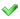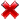### Using Assembler in Delphi

#### Table 3: Parameter Passing

This table gives an overview of how parameters are passed. A distinction is made between passing by value, declared as const or by reference. The "Value in register?" column indicates whether or not a specific type qualifies for passing in a register if it is passed as a value (applicable only to the "By Value" and "Const" columns!). Passing by reference in the form of a 32-bit pointer always qualifies for passing in a register. This is possible for all types, except method pointers, that are always passed via the stack. For example, an int64 type does not qualify according to the table below for passing in a register when it is passed as a value. However, since passing it by reference means passing a 32-bit pointer to the int64 value, passing an int64 by reference qualifies for use in a register.

 By Value Const Value inRegister? By Reference ShortInt 8-bit value(1) 8-bit value(1)32-bit pointer to 8-bit Value(1) SmallInt 16-bit value(1) 16-bit value(1)32-bit pointer to 16-bit Value(1) LongInt 32-bit value 32-bit value32-bit pointer to 32-bit Value Byte 8-bit value(1) 8-bit value(1)32-bit pointer to 8-bit Value(1) Word 16-bit value(1) 16-bit value(1)32-bit pointer to 16-bit Value(1) Dword 32-bit value 32-bit value32-bit pointer to 32-bit Value Int64 32-bit pointer to 64-bit value2 32-bit pointer to 64-bit value232-bit pointer to 64-bit Value2 Boolean 8-bit value(1) 8-bit value(1)32-bit pointer to 8-bit Value(1) ByteBool 8-bit value(1) 8-bit value(1)32-bit pointer to 8-bit Value(1) WordBool 16-bit value(1) 16-bit value(1)32-bit pointer to 16-bit Value(1) LongBool 32-bit value 32-bit value32-bit pointer to 32-bit Value AnsiChar 8-bit value(1) 8-bit value(1)32-bit pointer to 8-bit Value(1) WideChar 16-bit value(1) 16-bit value(1)32-bit pointer to 16-bit Value(1) ShortString 32-bit pointer to the string 32-bit pointer to the string32-bit pointer to the string AnsiString 32-bit pointer to the string 32-bit pointer to the string32-bit pointer to a 32-bit pointer to the string Variant 32-bit pointer to the variant 32-bit pointer to the variant32-bit pointer to the variant Pointer 32-bit pointer 32-bit pointer32-bit pointer to a 32-bit pointer Object reference 32-bit pointer to the object instance 32-bit pointer to the object instance32-bit pointer to a 32-bit pointer to the object instance Class reference 32-bit pointer to the class 32-bit pointer to the class32-bit pointer to a 32-bit pointer to the class Procedure pointer 32-bit pointer to the procedure/function 32-bit pointer to the procedure/function32-bit pointer to a 32-bit pointer to the procedure/function Method pointer 2x 32-bit pointer(3) 2x 32-bit pointer(3)2x 32-bit pointer(3) Set 8/16/32-bit value or 32-bit pointer(4) 8/16/32-bit value or 32-bit pointer(4)32-bit pointer to the set Record 8/16/32-bit value or 32-bit pointer(5) 8/16/32-bit value or 32-bit pointer(5)32-bit pointer to the record Static Array 8/16/32-bit value or 32-bit pointer(6) 8/16/32-bit value or 32-bit pointer(6)32-bit pointer to the array Dynamic Array 32-bit pointer to the array 32-bit pointer to the array32-bit pointer to a 32-bit pointer to the array Open Array 32-bit pointer to the array + 32-bit size value7 32-bit pointer to the array + 32-bit size value732-bit pointer to the array + 32-bit size value7 Single 32-bit value 32-bit value32-bit pointer to 32-bit Value Double (Real48) 64-bit value 64-bit value32-bit pointer to 64-bit Value Extended 80-bit value8 80-bit value832-bit pointer to 80-bit Value8 Currency 64-bit value 64-bit value32-bit pointer to 64-bit Value

(1) Data types that occupy less than 32-bits will still take up 32-bits. The actual value is stored in the lowest parts of the stack location or register and the content of the remaining part is undefined and should be treated as such at all times.
(2) The pointer points to the lowest dword of the value. The highest dword is stored in the next location.
(3) Method pointers are always passed on the stack. They consist of an instance pointer, which is pushed before the actual method pointer, which means the method pointer sits on the lowest address on the stack.
(4) If the Set contents fit into a byte/word/dword, its value is passed immediately, respectively as a 8/16/32 bit value. Otherwise, a 32-bit pointer to the set is passed.
(5) If the record contents fit into a byte/word/dword, the data is passed immediately, respectively as a 8/16/32 bit value. Otherwise, a 32-bit pointer to the record is passed.
(6) If the array contents fit into a byte/word/dword, the data is passed immediately, respectively as a 8/16/32 bit value. Otherwise, a 32-bit pointer to the array is passed.
(7) Open arrays are passed as 2 parameters: the first one is the pointer to the actual array, the second one is the number of elements in the array. As such, passing an open array parameter actually occupies 2 parameter slots. For instance: if you use the `register` calling convention and you pass one open array parameter `eax` will contain the pointer to the array and `edx` will contain the number of elements. See Chapter 2 for details about calling conventions. Also note that open array parameters reside on the stack, so refrain from using very large arrays.
(8) While the value itself occupies only 10 bytes, 12 bytes are actually allocated (3 dwords). The content of the last 2 bytes should be considered undefined.